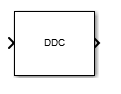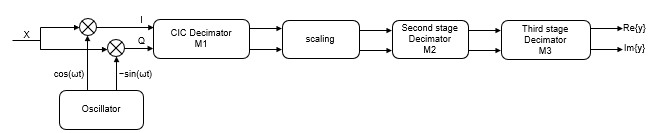Main Content

# Digital Down-Converter

Translate digital signal from Intermediate Frequency (IF) band to baseband and decimate it

## Library

Signal Operations

`dspsigops`

•## Description

The Digital Down-Converter (DDC) block converts a digitized real signal, centered at an intermediate frequency (IF) to a baseband complex signal centered at zero frequency. The DDC block downsamples the frequency down-converted signal using a cascade of three decimation filters. This block designs the decimation filters according to the filter parameters set in the block dialog box.

## Structure

This block brings the capabilities of `dsp.DigitalDownConverter` System object™ to the Simulink® environment.

The DDC block consists of a CIC decimator, a CIC compensator, and a FIR decimator. You can bypass the FIR Decimator, depending on how you set the DDC block parameters.For more information on the structure that the DDC block uses, including the flow of fixed-point input, see the Creation section in `dsp.DigitalDownConverter`.

## Dialog Box

### Main TabDecimation factor

Decimation factor, specified as a positive integer scalar, or as a 1-by-2 or 1-by-3 vector of positive integers. The default is `100`.

When you set this parameter to a scalar, the block chooses the decimation factors for each of the three filtering stages.

When you set this parameter to a 1-by-2 vector, the block bypasses the third filter stage and sets the decimation factor of the first and second filtering stages to the values in the first and second vector elements, respectively. Both elements of Decimation factor must be greater than `1`.

When you set this parameter to a 1-by-3 vector, the ith element of the vector specifies the decimation factor for the ith filtering stage. The first and second elements of Decimation factor must be greater than `1`, and the third element must be `1` or `2`.

Minimum order filter design

When you select this check box, the block designs filters with the minimum order that meets the requirements specified in these parameters:

• Passband ripple of cascade response (dB)

• Stopband attenuation of cascade response (dB)

• Two sided bandwidth of input signal (Hz)

• Source of stopband frequency

• Stopband frequency (Hz)

When you clear this check box, the block designs filters with orders that you specify in Number of sections of CIC decimator, Order of CIC compensation filter stage, and Order of third filter stage. The filter designs meet the passband and stopband frequency specifications that you set in Two sided bandwidth of input signal (Hz), Source of stopband frequency, and Stopband frequency (Hz). By default, this check box is selected.

Number of sections of CIC decimator

Number of sections in the CIC decimator, specified as a positive integer scalar. This parameter applies when you clear the Minimum order filter design check box. The default is `3`.

Order of CIC compensation filter stage

Order of the CIC compensation filter stage, specified as a positive integer scalar. This parameter applies when you clear the Minimum order filter design check box. The default is `12`.

Order of third filter stage

Order of the third filter stage, specified as an even positive integer scalar. When you specify Decimation factor as a 1-by-2 vector, the block ignores the value of Order of third filter stage because the block bypasses the third filter stage. This parameter applies when you clear the Minimum order filter design check box. The default is `10`.

Two sided bandwidth of input signal (Hz)

Two sided bandwidth of the input signal, specified as a positive integer scalar. The block sets the passband frequency of the cascade of filters to half the value that you specify in this parameter. Set the value of this parameter to less than Input sample rate/Decimation factor. When you select the Inherit sample rate from input check box, then set this value to less than ((`1`/Ts) / Decimation factor), where Ts is the sample time of the input signal. The default is `200` kHz.

Source of stopband frequency

Source of the stopband frequency, specified as `Auto` or `Property`. The default is `Auto`.

When you set this parameter to `Auto`, the block places the cutoff frequency of the cascade filter response at approximately Fc = SampleRate / M/2 Hz, where M is the total decimation factor specified in Decimation factor. SampleRate is computed as `1`/ Ts, where Ts is the sample time of the input signal. The block computes the stopband frequency as Fstop = Fc + (TW / 2). TW is the transition bandwidth of the cascade response, computed as 2×(FcFp), where the passband frequency, Fp, equals Bandwidth/2.

When you set this parameter to `Property`, specify the source in Stopband frequency (Hz).

Stopband frequency (Hz)

Stopband frequency, specified as a double-precision positive scalar. This parameter applies when you set the Source of stopband frequency to `Property`. The default is `150` kHz.

Passband ripple of cascade response (dB)

Passband ripple of the cascade response, specified as a double-precision positive scalar. When you select the Minimum order filter design, the block designs the filters so that the cascade response meets the passband ripple that you specify in Passband ripple of cascade response (dB). This parameter applies when you select the Minimum order filter design. The default is `0.1` dB.

Stopband attenuation of cascade response (dB)

Stopband attenuation of the cascade response, specified as a double-precision positive scalar. When you select the Minimum order filter design, the block designs the filters so that the cascade response meets the stopband attenuation that you specify in Stopband attenuation of cascade response (dB). This parameter applies when you select the Minimum order filter design. The default is `60`.

Type of oscillator

Oscillator type, specified as one of the following:

• `Sine wave` (default) — The block performs frequency down conversion on input signal using a complex exponential obtained from samples of a sinusoidal trigonometric function.

• `NCO` — The block performs frequency down conversion on input signal with a complex exponential obtained using a numerically controlled oscillator (NCO).

• `Input port` — The block performs frequency down conversion on input signal using the complex signal that you provide through the input port of the block.

• `None` — The mixer stage in the block is not present and the block acts as three stage cascaded decimator.

Center frequency of input signal (Hz)

Center frequency of the input signal, specified as a double-precision positive scalar that is less than or equal to half the sample rate. The block downconverts the input signal from the passband center frequency, which you specify in Center frequency of input signal (Hz), to `0` Hz. This parameter applies when you set Type of oscillator to ```Sine wave``` or `NCO`. The default is `14e6`.

Number of NCO accumulator bits

Number of NCO accumulator bits, specified as an integer scalar in the range `[1 128]`. This parameter applies when you set Type of oscillator to `NCO`. The default is `16`.

Number of NCO quantized accumulator

Number of NCO quantized accumulator bits, specified as an integer scalar in the range `[1 128]`. This value must be less than the value you specify in Number of NCO accumulator bits. This parameter applies when you set Type of oscillator to `NCO`. The default is `12`.

Dither control for NCO

When you select this check box, a number of dither bits specified in Number of NCO dither bits applies dither to the NCO signal. This parameter applies when you set Type of oscillator to `NCO`. By default, this check box is selected.

Number of NCO dither bits

Number of NCO dither bits, specified as an integer scalar smaller than the number of accumulator bits that you specify in Number of NCO accumulator bits. This parameter applies when you set Type of oscillator to `NCO` and select the Dither control for NCO. The default is `4`.

Inherit sample rate from input

When you select this check box, sample rate is computed as `N`/ Ts, where N is the frame size of the input signal, and Ts is the sample time of the input signal. When you clear this check box, the block’s sample rate is the value specified in Input sample rate (Hz). By default, this check box is selected.

Input sample rate

Input sample rate, specified as a positive scalar value, greater than or equal to twice the value of the Center frequency of input signal (Hz). The default is `30` MHz. This parameter applies when you clear the Inherit sample rate from input check box.

View Filter Response

Opens the Filter Visualization Tool FVTool and displays the magnitude/phase response of each stage as well as the cascade of stages in the Digital Down-Converter. The response is based on the block dialog box parameters. Changes made to these parameters update FVTool.To update the magnitude response while FVTool is running, modify the dialog box parameters and click .

Simulate using

Type of simulation to run. You can set this parameter to:

• `Code generation` (default)

Simulate model using generated C code. The first time you run a simulation, Simulink generates C code for the block. The C code is reused for subsequent simulations, as long as the model does not change. This option requires additional startup time but provides faster simulation speed than ```Interpreted execution```.

• `Interpreted execution`

Simulate model using the MATLAB®  interpreter. This option shortens startup time but has slower simulation speed than ```Code generation```.

### Data Types tabStage input

Data type of the input of the first, second, and third filter stages. You can set this parameter to:

• `Inherit: Same as input` (default) — The block inherits the Stage input from the input signal.

• `fixdt([],16,0)` — Fixed-point data type with binary point scaling. Specify the sign mode of this data type as `[]` or `true`.

• An expression that evaluates to a data type, for example, `numerictype([],16,15)`. Specify the sign mode of this data type as `[]` or `true`.

Click the buttonto display the data type assistant, which helps you set the stage input parameter.

See Specify Data Types Using Data Type Assistant (Simulink) for more information.

Output

Data type of the block output. You can set this parameter to:

• `Inherit: Same as input` (default) — The block Inherits the output datatype from the input.

• `fixdt([],16,0)` — Fixed-point data type with binary point scaling. Specify the sign mode of this data type as `[]` or `true`.

• An expression that evaluates to a data type, for example, `numerictype([],16,15)`. Specify the sign mode of this data type as `[]` or `true`.

Click the buttonto display the data type assistant, which helps you set the Output parameter.

See Specify Data Types Using Data Type Assistant (Simulink) for more information.

Minimum

Minimum value of the block output. The default value is `[]` (unspecified). Simulink software uses this value to perform:

• Simulation range checking (see Specify Signal Ranges (Simulink))

• Automatic scaling of fixed-point data types

Maximum

Maximum value of the block output. The default value is `[]` (unspecified). Simulink software uses this value to perform:

• Simulation range checking (see Specify Signal Ranges (Simulink))

• Automatic scaling of fixed-point data types

Lock data type settings against changes by the fixed-point tools

Select this parameter to prevent the fixed-point tools from overriding the data types you specify on the block mask.

## Supported Data Types

PortSupported Data Types

Input

• Double-precision floating point

• Single-precision floating point

• Fixed point (signed only)

• 8-, 16-, 32- and 64-bit signed integers

Output

• Double-precision floating point

• Single-precision floating point

• Fixed point (signed only)

• 8-, 16-, 32- and 64-bit signed integers

## See Also

### Blocks

Introduced in R2015a

Watch now